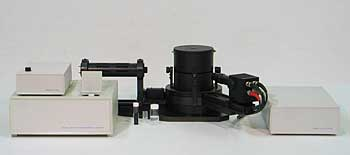# DYNAMIC LIGHT SCATTERING (PHOTON CORRELATION SPECTROSCOPY)

The photon-correlation spectrometers IKO Sizer are based on the Photon Correlation Spectroscopy (PCS) technique and designed for measurements of sub-micron particle sizes, diffusion coefficients, viscosities, molecular weights of polymers in basic and applied studies. The PCS method consists in determining the velocity distribution of particles movement by measuring dynamic fluctuations of intensity of scattered light. The disperse particles or macromolecules suspended in a liquid medium undergo Brownian motion which causes the fluctuations of the local concentration of the particles, resulting in local inhomogeneities of the refractive index. This in turn results in fluctuations of intensity of the scattered light. The linewidth of the light scattered spectrum G (defined as the half-width at half-maximum) is proportional to the diffusion coefficient of the particles D (Eq.1):

gDk2 where k4pn is the refractive index of the medium, A the laser wavelength, and H the scattering angle. With the assumption that the particles are spherical and non-interacting, the mean radius is obtained from the Stokes-Einstein equation:

RkbT where kB is the Boltzmann constant, T the temperature, and N the shear viscosity of the solvent. Information about the light-scattering spectrum can be obtained from the autocorrelation function Gt of the light-scattering intensity. For the simplest case of spherical monodisperse non-interacting particles in a dust-free fluid, the characteristic decay time of the correlation function is inversely proportional to the linewidth of the spectrum. Therefore, the diffusion coefficient and either particle size or viscosity can be found by fitting the measured correlation function to a single exponential function. A characteristic autocorrelation function of the scattered light is shown below where the baseline b is proportional to the total intensity I, and it can be determined experimentally.
Gt there exist two techniques of measuring the correlation function: heterodyning and homodyning. In heterodyne measurements, which are most suitable for small intensities, the scattered light is mixed coherently with a static light source at the incident wavelength, and the static field is added to the scattered fields at the photodetector. Eq.1 that connects the linewidth and the diffusion coefficient D is given for a heterodyne spectrum. In homodyne measurements the photodetector receives scattered light only. Homodyning is most suitable for strong intensities (e.g. near the critical point of the fluid, or for colloid systems). For a homodyne spectrum the connection between G and D reads:

### PRINCIPLE OF OPERATION AND SETUP ARRANGEMENT

G2Dk2 he PCS method consists in analyzing the velocity distribution of particles movement by measuring dynamic fluctuations of light scattering intensity:

1. The disperse particles or macromolecules suspended in a liquid medium undergo Brownian motion which causes fluctuations of local concentration of the particles, resulting in local inhomogeneities of the refractive index. This in turn results in fluctuations of intensity of the scattered light.
2. The diffusion coefficient of the particles is inversely proportional to the decay time of light scattering fluctuations. The decay time is obtained from the time-dependent correlation function of the scattered light.
3. The particle size is calculated in accordance with Stokes-Einstein formula relating the particle size to the diffusion coefficient and viscosity.